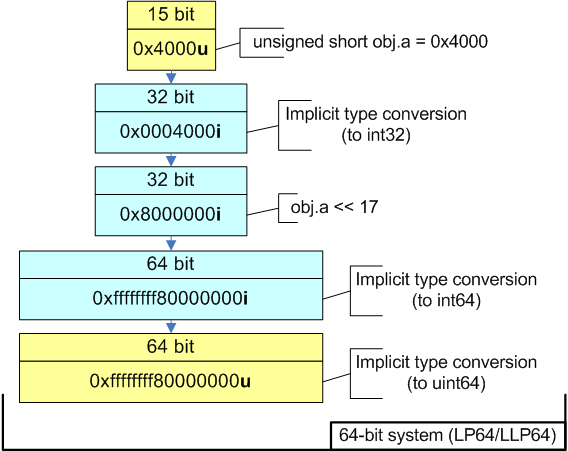﻿ Lesson 11. Pattern 3. Shift operations# Lesson 11. Pattern 3. Shift operations

It is easy to make a mistake in code that works with separate bits. The pattern of 64-bit errors under consideration relates to shift operations. Here is an example of code:

``````ptrdiff_t SetBitN(ptrdiff_t value, unsigned bitNum) {
ptrdiff_t mask = 1 << bitNum;
}``````

This code works well on a 32-bit architecture and allows you to set a bit with numbers from 0 to 31 into one. After porting the program to a 64-bit platform you need to set bits from 0 to 63. But this code will never set the bits with the numbers 32-63. Note that the numerical literal "1" has int type and causes an overflow when a shift in 32 positions occurs as shown in Figure 1. As a result, we will get 0 (Figure 1-B) or 1 (Figure 1-C) depending on the compiler implementation.

Figure 1 - a) Correct setting of the 31-st bit in a 32-bit code; b,c) - Incorrect setting of the 32-nd bit on a 64-bit system (two variants of behavior)

To correct the code we must make the type of the constant "1" the same as that of mask variable:

``ptrdiff_t mask = ptrdiff_t(1) << bitNum;``

Note also that the non-corrected code will lead to one more interesting error. When setting the 31-st bit on a 64-bit system, the function's result will be the value 0xffffffff80000000 (see Figure 2). The result of the expression 1 << 31 is the negative number -2147483648. This number is presented in a 64-bit integer variable as 0xffffffff80000000.

Figure 2 - The error of setting the 31-st bit on a 64-bit system.

You should remember and take into consideration the effects of shifting values of different types. To better understand all said above, consider some interesting expressions with shifts in a 64-bit system shown in Table 1.

Table 1 - Expressions with shifts and their results in a 64-bit system (we used Visual C++ 2005 compiler)

The type of errors we have described is considered dangerous not only from the viewpoint of program operation correctness but from the viewpoint of security as well. Potentially, by manipulating with the input data of such incorrect functions one can get inadmissible rights when, for example, dealing with processing of access permissions' masks defined by separate bits. Questions related to exploiting errors in 64-bit code for application cracking and compromise are described in the article "Safety of 64-bit code".

Now a subtler example:

``````struct BitFieldStruct {
unsigned short a:15;
unsigned short b:13;
};
BitFieldStruct obj;
obj.a = 0x4000;
size_t addr = obj.a << 17; //Sign Extension
//Output on 32-bit system: 0x80000000
//Output on 64-bit system: 0xffffffff80000000``````

In the 32-bit environment, the order of calculating the expression will be as shown in Figure 3.Figure 3 - Calculation of expression in 32-bit code

Note that a sign extension of "unsigned short" type to "signed int" takes place when calculating "obj.a << 17". To make it clear, consider the following code:

``````#include <stdio.h>
template <typename T> void PrintType(T)
{
printf("type is %s %d-bit\n",
(T)-1 < 0 ? "signed" : "unsigned", sizeof(T)*8);
}
struct BitFieldStruct {
unsigned short a:15;
unsigned short b:13;
};
int main(void)
{
BitFieldStruct bf;
PrintType( bf.a );
PrintType( bf.a << 2);
return 0;
}
Result:
type is unsigned 16-bit
type is signed 32-bit``````

Now let us see the consequence of the sign extension in a 64-bit code. The sequence of calculating the expression is shown in Figure 4.Figure 4 - Calculation of expression in 64-bit code

The member of "obj.a" structure is converted from the bit field of "unsigned short" type to "int". "obj.a << 17" expression has "int" type but it is converted to ptrdiff_t and then to size_t before it is assigned to addr variable. As a result, we will get the value 0xffffffff80000000 instead of 0x0000000080000000 expected.

Be careful when working with bit fields. To avoid the situation described in our example we need only to explicitly convert "obj.a" to size_t type.

``````...
size_t addr = size_t(obj.a) << 17;
//Output on 32-bit system: 0x80000000
//Output on 64-bit system: 0x80000000``````

## Diagnosis

Potentially unsafe shifts are detected by PVS-Studio static analyzer when it detects an implicit extension of a 32-bit type to memsize type. The analyzer will warn you about the unsafe construct with the diagnostic warning V101. The shift operation is not suspicious by itself. But the analyzer detects an implicit extension of int type to memsize type when it is assigned to a variable, and informs the programmer about it to check the code fragment that may contain an error. Correspondingly, when there is no extension, the analyzer considers the code safe. For example: "int mask = 1 << bitNum;".

The course authors: Andrey Karpov (karpov@viva64.com), Evgeniy Ryzhkov (evg@viva64.com).

The rightholder of the course "Lessons on development of 64-bit C/C++ applications" is OOO "Program Verification Systems". The company develops software in the sphere of source program code analysis. The company's site: http://www.viva64.com.##### Use PVS-Studio to search for bugs in C, C++, C# and Java

We offer you to check your project code with PVS-Studio. Just one bug found in the project will show you the benefits of the static code analysis methodology better than a dozen of the articles.

410
14 111

### Do you make errors in the code?